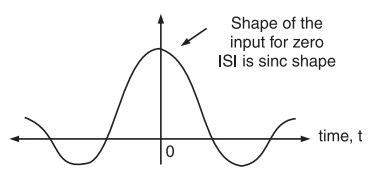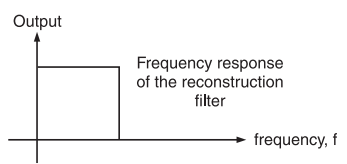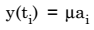### Short Questions and Answers on Digital Data Transmission

Q.1. What is Baseband binary data transmission system?

Ans. The data transmission system which makes use of a baseband channel for transmitting a binary data is known as baseband data transmission i.e., the system using a channel whose bandwidth is equal to the bandwidth of the message signal, called a baseband channel is referred to as baseband data transmission system.

Q.2. What is a Baseband binary PAM system? Explain.

Ans. Pulse amplitude modulation scheme is a system in which the amplitude of discrete pulses (carrier) vary along with the message signal. Thus, baseband binary PAM system is the scheme in which the amplitude transmitted pulses take any one of the two amplitude levels i.e., either 1 (or) 0.

Q.3. What is the drawback of binary PAM system?

Ans. (i) The bandwidth required for baseband binary PAM is approximately rb /2 Hz, in order to transmit data at a rate of rb bits/sec, with zero ISI.

(ii) If the bandwith available is exactly rb /2 Hz, then the binary PAM system transmits data at a rate rb bits/sec only if it uses an ideal low pass filter at the transmitter and receiver.

(iii) Thus, such filters are physically unrealizable and if any systems are approximated to these filters then those systems are extremely sensitive to the perturbations (or) disturbances in rate, timing (or) channel characteristics. This is the drawback of baseband binary PAM system.

Q.4. What do you mean by an Eye Diagram?

Ans. The performance of baseband PAM system depends on the amount of ISI and channel noise. The distribution of ISI and channel noise in the system can be readily observed by displaying the received waveform Y(t) on an oscilloscope using a sweep rate that is a fraction of the symbol rate rs. The resulting display shape resembles a human eye and is widely known as the Eye pattern of the system.

Q.5. What is Equalization?

Ans. The process of correcting channel induced distortion is called equalization.

Q.6. What is the effect of ISI? Explain.

Ans. Following are the effects of ISI:

(i) In the absence of ISI and noise, the transmitted bit can be decoded correctly at the receiver.

(ii) The presence of ISI will introduce errors in the decision at the receiver output.

(iii) Hence, the receiver can make an error in deciding whether it has received a logic 1 or a logic 0.

Q.7. What is remedy to Reduce ISI?

Ans. Remedy to Reduce ISI are given below :

(i) It has been proved that the function which produces a zero intersymbol interference is a sinc function. Hence, instead of a rectangular pulse if we transmit a sinc pulse then the ISI can be reduced to zero.

(ii) This is known as Nyquist Pulse Shaping. The sinc pulse transmitted to have a zero ISI shown in figure.1.Fig.1 : Ideal pulse shape for zero ISI

(iii) Further, we know that Fourier transform of a sinc pulse is a rectangular function. Hence, to preserve all the frequency components, the frequency response of the filter must be exactly flat in the passband and zero in the attenuation band as shown in figure.2.Fig.2 : Frequency Response of the filter

Q.8. What is Nyquist’s criterion for distortionless transmission? Explain.

Ans. We have observed that in absence of the ISI, we haveThis expression shows that under these conditions, the ith transmitted bit can be decoded correctly.

In order to minimize the effects of ISI, we have to design the transmitting and receiving filters properly.

The transfer function of the channel and the shape of transmitted pulse are generally specified.

Therefore, it becomes the first step towards design of filters.

From this information we have to determine the transfer functions of the transmitting and receiving filters, to reconstruct the transmitted data sequence {bk}. This is achieved by first extracting and then decoding the corresponding sequence of weights from the output y(t).

Q.9. What is the meaning of word ‘RZ’.

Ans. RZ means return to zero. In RZ formats, the code signal reduces to zero after a duration of Tb / 2 seconds.

Q.10. How can we improve the synchronizing capacity of a code?

Ans. By introducing zero crossings at regular intervals of time.

Q.11. What is intersymbol interference (ISI).

Ans. Intersymbol interference (ISI) is the signal distortion which is introduced when a pulsed waveform is transmitted over a bandlimited channel.

Q.12. What is the remedy to ISI?

Ans. The remedy to ISI  is Nyquist pulse shaping.

Q.13. What is the shape of transmitted pulse to avoid ISI?

Ans. Shape of transmitted pulse should be ‘sinc pulse’.

Q.14. How can we measure ISI on CRO?

Ans. We can measure ISI on CRO with the help of eye diagram.

Q.15. What is the significance of eye opening?

Ans. The width of the eye opening defines the interval over which the received wave can be sampled without any error due to ISI.

Q.16. What is the effect of ISI?

Ans. Effect of ISI is introduction of errors in the received signal.